Enhancement of Voltage Stability by using FACTS under Normal andamp; Post-Fault Steady State Conditions | OMICS International
Journal of Electrical & Electronic Systems

# Enhancement of Voltage Stability by using FACTS under Normal & Post-Fault Steady State Conditions

K. Venkata Ramana Reddy, M. Padma Lalitha* and P. Harsha Vardhan Reddy

Department of EEE, Annamacharya Institute of Technology and sciences, Rajampet, Kadapa (DT), AP (ST), India

*Corresponding Author:
Department of EEE, Annamacharya Institute of Technology and sciences
Rajampet, Kadapa (DT), AP (ST), India
E-mail: [email protected]

Received Date: August 21, 2013; Accepted Date: September 20, 2013; Published Date: September 23, 2013

Citation: Venkata Ramana Reddy. K, Padma Lalitha. M, Harsha Vardhan Reddy. P (2013) Enhancement of Voltage Stability by using FACTS under Normal & Post-Fault Steady State Conditions. J Electr Electron Syst 2:113. doi:10.4172/2332-0796.1000113

Copyright: © 2013 Venkata Ramana Reddy K, et al. This is an open-access article distributed under the terms of the Creative Commons Attribution License, which permits unrestricted use, distribution, and reproduction in any medium, provided the original author and source are credited.

Visit for more related articles at Journal of Electrical & Electronic Systems

#### Abstract

In this paper an IEEE standard test system has been considered and Load flows were computed by using Newton- Raphson method with the help of MATLAB and a weak bus is identified. The bus that is with a low voltage magnitude is incorporated with FACTS devices namely TCSC and SVC in order to improve the voltage under normal conditions. Then the reactive power at a particular bus is increased until it reaches to the instability point and the voltage stability condition is evaluated by using an L-index method. The values which approach unity imply that it reaches to instability, and corresponding bus can be treated as weak bus i.e. with a highest value of L-index, and is incorporated with FACTS devices to enhance the voltage stability under post-fault steady state condition also.

#### Keywords

L-index, SVC; TCSC; MATLAB; Post-fault steady state condition

#### Introduction

As a result of ever-increasing demand of electric power, the electricity supply industry is undergoing profound transformation worldwide. This makes the existing power transmission system highly complex. To meet the increasing demand of electricity in a power system it is essential to increase the transmitted power either by installing new transmission lines or by improving the existing transmission lines by adding new devices. Installation of new transmission lines in a power system leads to the technological complexities such as economic and environmental considerations that includes cost, delay in construction as so on. Flexible Alternating Current Transmission System (FACTS) technology gave up new ways for controlling power flows and enhancing the usable capacity of transmission lines. FACTS are system comprised of static equipment used for the AC transmission of electrical energy. It is meant to enhance controllability and increasing the power transfer capability of the power system network. The concept of FACTS was first defined by Hingorani . It usually refers to the application of high power semi conductor devices to control different parameters and electrical variables such as voltage, impedance, and phase angle, current, active and reactive power. This paper addresses the static modeling of Static Var Compensator (SVC) and Thyristor Controlled Series Compensator (TCSC), and their capabilities to improve the voltage profile by using MATLAB code.

#### Voltage Stability Index

In order to prevent the occurrence of voltage collapse, it is essential to accurately predict the operating condition of a power system. Kessel  developed a voltage stability index based on the solution of the power flow equation. The L-index is a quantitative measure for the estimation of the distance of actual state of the system stability limit.

The L- index describes the stability of the complete system. A load flow result is obtained for a given system operating condition which is otherwise available from the output of an on line estimator. The load flow algorithm incorporates load characteristics and generator control characteristics. For an n-bus power system, buses can be separated into two groups: Bring all load buses to the head and denote them as α L and put the PV buses the tail and term them as αG i.e., αL ={1,2,...,n L} and αG ={ nL+1, nL+2,......n-1, n}

Where, nL is the number of load buses.

The following hybrid system equation is then obtained: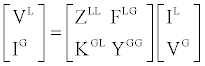(1)

where,

ZLL, FLG, KGL, and YGG are sub-block of matrix H;

VG, IG, VL, IL are voltage and current vector of PV buses and load buses.

Voltage stability index Lj for any load bus can be defined as given below.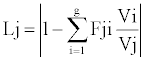(2)

#### Static Var Compensator

SVC can be defined as a shunt connected static var generator or absorber whose output is adjusted to exchange capacitive or inductive current so as to maintain or control specific parameters of the electrical power system (typically bus voltage).

SVCs are primarily used in power systems for voltage control or for improving system stability. The advanced models depart from the conventional generator-type representation of the SVC and are based instead on the variable shunt susceptance concept. In the latter case, the SVC state variables are combined with the nodal voltage magnitudes and angles of the network in a single frame of reference for unified, iterative solutions using the Newton-Raphson method.

In practice the SVC can be seen as an adjustable reactance with either firing-angle limits or reactance limits.

The equivalent circuit is used to derive the SVC nonlinear power equations and the linearised equations required by Newton’s method (Figure 1).

The linearised equation is given by,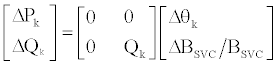(3)

At the end of iteration (i), the variable shunt susceptance BSVC is updated as follows,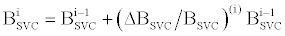(4)

#### Thyristor Controlled Series Compensator

A TCSC can be defined as a capacitive reactance compensator which consists of a series capacitor bank shunted by a thyristorcontrolled reactor in order to provide a smoothly variable series capacitive reactance. The basic conceptual TCSC module comprises a series capacitor, C, in parallel with a thyristor-controlled reactor, LS, as shown in Figure 2.

The TCSC power flow model presented in this section is based on the simple concept of a variable series reactance, the value of which is adjusted automatically to constrain the power flow across the branch to a specified value. The amount of reactance is determined efficiently using Newton’s method. The changing reactance XTCSC represents the equivalent reactance of all the seriesconnected modules making up the TCSC, when operating in either the inductive or the capacitive regions.

The transfer admittance matrix of the variable series compensator is given by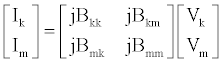(5)

For the power equations at bus m, the subscripts k and m are exchanged and the active and reactive power equations at bus k are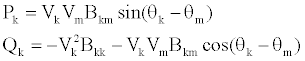(6)

#### Results and Discussions

A MATLAB code for both techniques was developed for the validation of the proposed FACTS, and it is tested on IEEE 5-Bus test system and the following observations were made by using Newton- Raphson method under normal and transient conditions.

Case I: Normal condition

After running the power flow by using Newton-Raphson method on an IEEE standard test system (5-bus), a weak bus is identified (Figure 3). Under normal condition with SVC it is found that the voltage profile at bus 5 where SVC is connected is improved.

Initially under normal condition without SVC the voltage magnitude in per unit (pu) at bus 5 is 0.9776, and the L-index of corresponding bus 5 is 0.0328, but after placing SVC, voltage magnitude improved to 1.000 pu and the L-index of corresponding bus 5 is 0.0099. Similarly after placing TCSC, the voltage profile at bus 5 is also improved to 0.9789 pu as shown in Table 1. The L- index value of each load bus is performed as shown in Table 2.

Bus no Voltage magnitude without SVC (OR) TCSC Voltage magnitude with SVC Voltage magnitude with  TCSC
1 1.06 1.06 1.06
2 1 1 1
3 0.9925 0.9956 0.9966
4 0.9894 0.9934 0.9924
5 0.9776 1 0.9789
6     0.9784

Table 1: Under Normal condition.

Bus no L-index Without SVC (or) TCSC L-index With SVC L-index With TCSC
3 0.0299 0.0262 0.0296
4 0.0304 0.0267 0.0324
5 0.0328 0.0099 0.0234
6 ------ ------- 0.0536

Table 2: L-indices.

Case II: Post-fault condition

Then by changing reactive power at bus 5, i.e. from Q = 0.05 to Q = 0.2 MVAR, a weak bus is identified as bus 5 and it is incorporated by SVC and TCSC in order to improve the voltage profile as shown in Table 3.

Bus no Voltage magnitude without SVC (OR) TCSC Voltage magnitude with    SVC Voltage magnitude with  TCSC
1 1.06 1.06 1.06
2 1 1 1
3 0.9899 0.9967 0.99
4 0.986 0.9948 0.9856
5 0.9639 1 0.9641
6 ----------- ----------- 0.9631

Table 3: Under Post-fault condition.

The L-index of each load bus was performed with and without SVC as well as TCSC under post fault condition as shown in Table 4.

Bus no L-index Without SVC (or) TCSC L-index With SVC L-index With TCSC
3 0.0326 0.0255 0.0365
4 0.0338 0.0247 0.0396
5 0.0475 0.0099 0.0391
6 ---------   0.0702

Table 4: L-indices.

#### Conclusion

This paper depicts that the improved voltage profile of the power system under Normal and Transient conditions with the incorporation of Static VAR compensator (SVC) and Thyristor Controlled Series Compensator (TCSC). SVC and TCSC were able to regulate the bus voltage magnitude at which it is connected over its full range of operation when there is a need.

The optimal location is identified by using L-index method. This can also be performed by using Fast decoupled method to analyze the voltage stability due to change in real power.

#### References

Select your language of interest to view the total content in your interested language

### Article Usage

• Total views: 13068
• [From(publication date):
December-2013 - Jan 20, 2020]
• Breakdown by view type
• HTML page views : 9193Can't read the image? click here to refresh